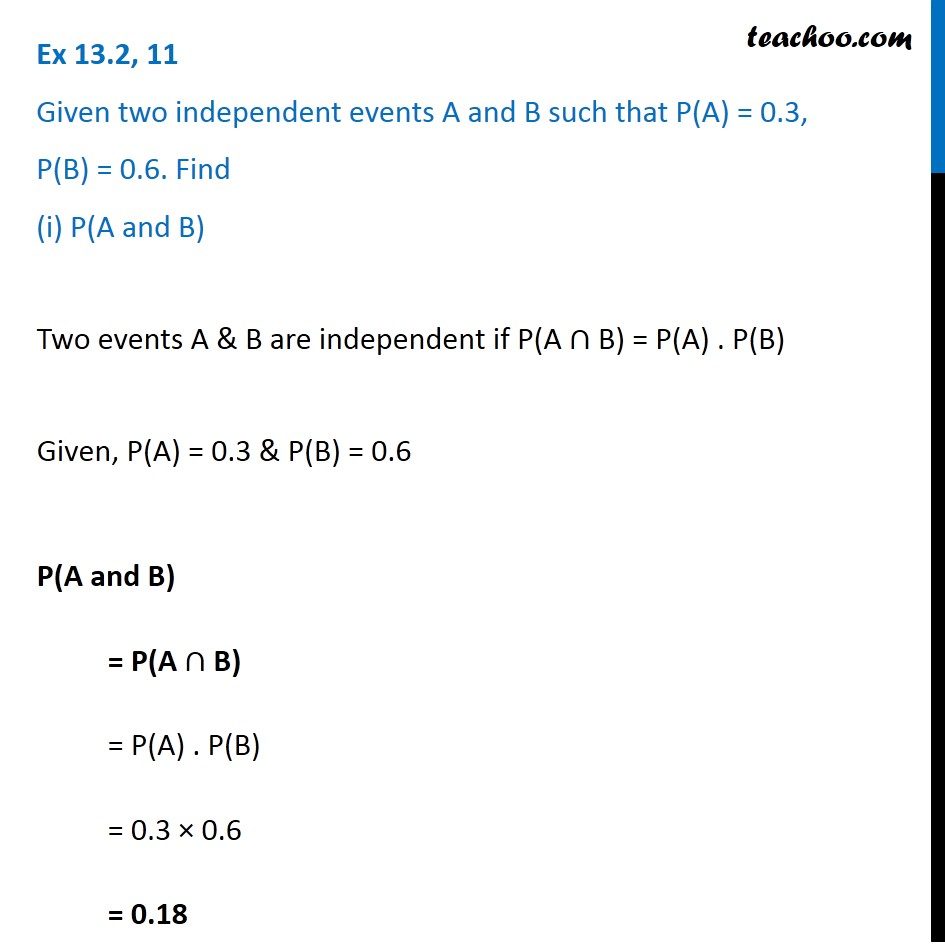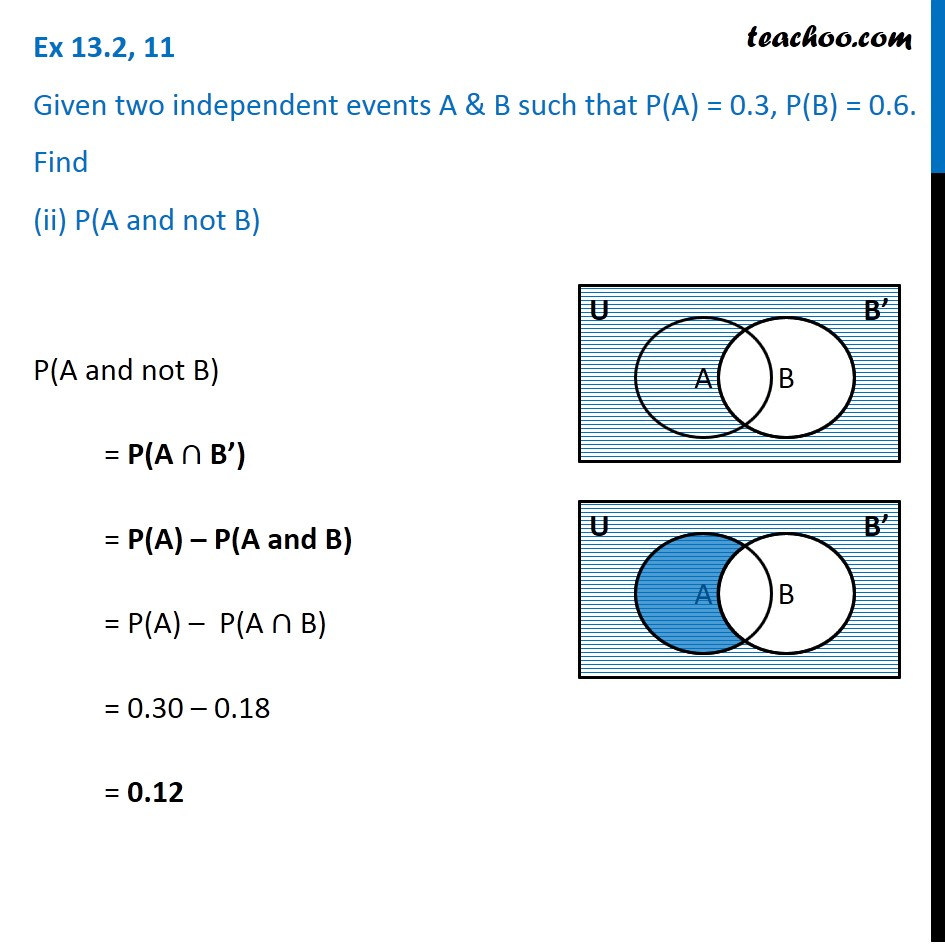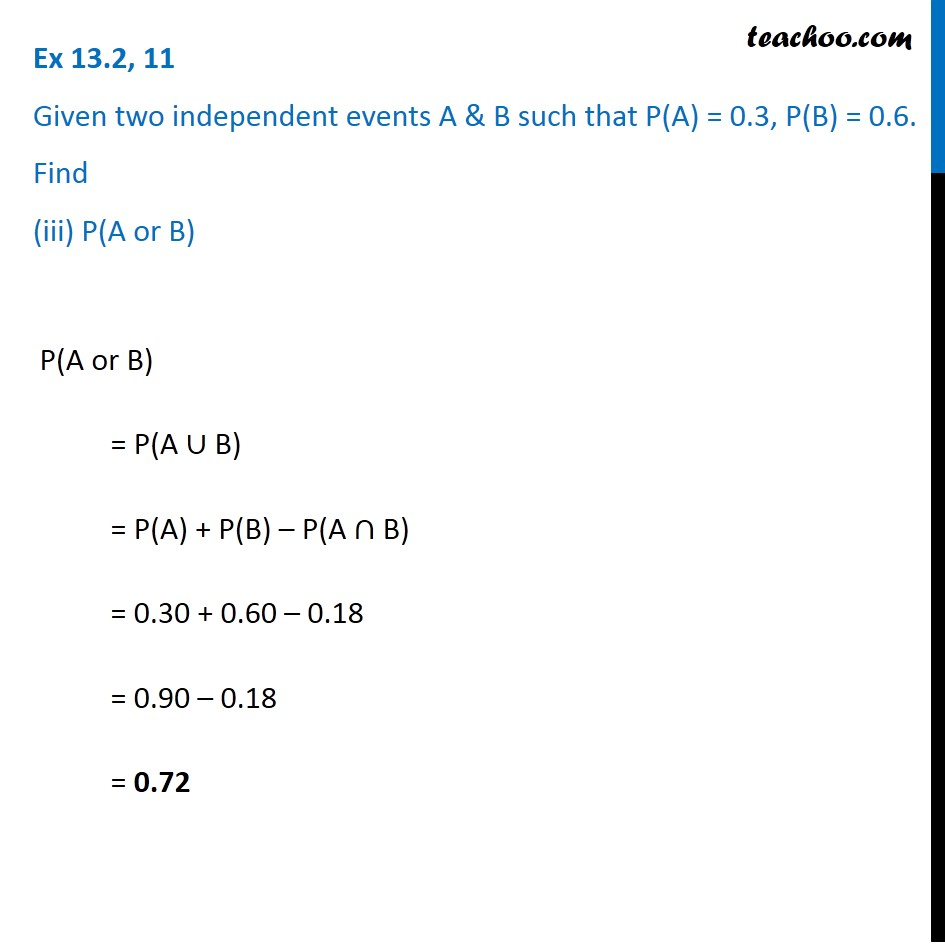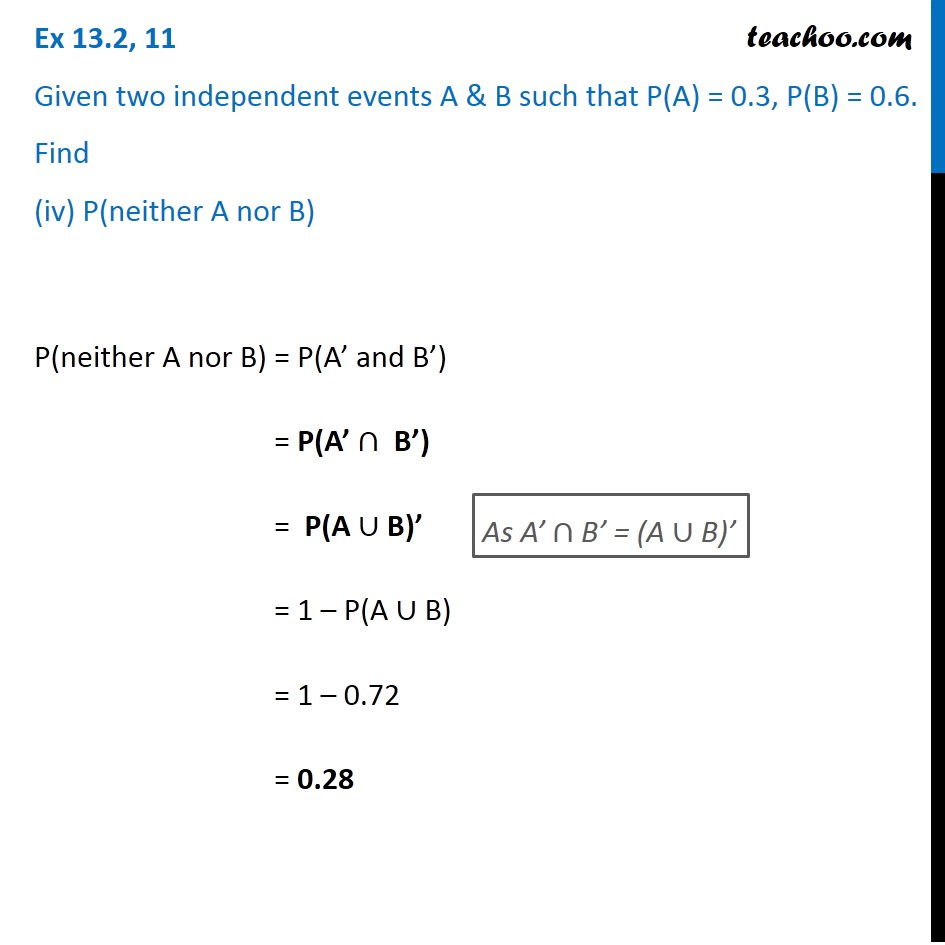Subscribe to our Youtube Channel - https://you.tube/teachoo

1. Chapter 13 Class 12 Probability
2. Serial order wise
3. Ex 13.2

Transcript

Ex 13.2, 11 Given two independent events A and B such that P(A) = 0.3, P(B) = 0.6. Find (i) P(A and B) Two events A & B are independent if P(A ∩ B) = P(A) . P(B) Given, P(A) = 0.3 & P(B) = 0.6 P(A and B) = P(A ∩ B) = P(A) . P(B) = 0.3 × 0.6 = 0.18 Ex 13.2, 11 Given two independent events A & B such that P(A) = 0.3, P(B) = 0.6. Find (ii) P(A and not B) P(A and not B) = P(A ∩ B’) = P(A) – P(A and B) = P(A) – P(A ∩ B) = 0.30 – 0.18 = 0.12 Ex 13.2, 11 Given two independent events A & B such that P(A) = 0.3, P(B) = 0.6. Find (iii) P(A or B)P(A or B) = P(A ∪ B) = P(A) + P(B) – P(A ∩ B) = 0.30 + 0.60 – 0.18 = 0.90 – 0.18 = 0.72 Ex 13.2, 11 Given two independent events A & B such that P(A) = 0.3, P(B) = 0.6. Find (iv) P(neither A nor B) P(neither A nor B) = P(A’ and B’) = P(A’ ∩ B’) = P(A ∪ B)’ = 1 – P(A ∪ B) = 1 – 0.72 = 0.28

Ex 13.2